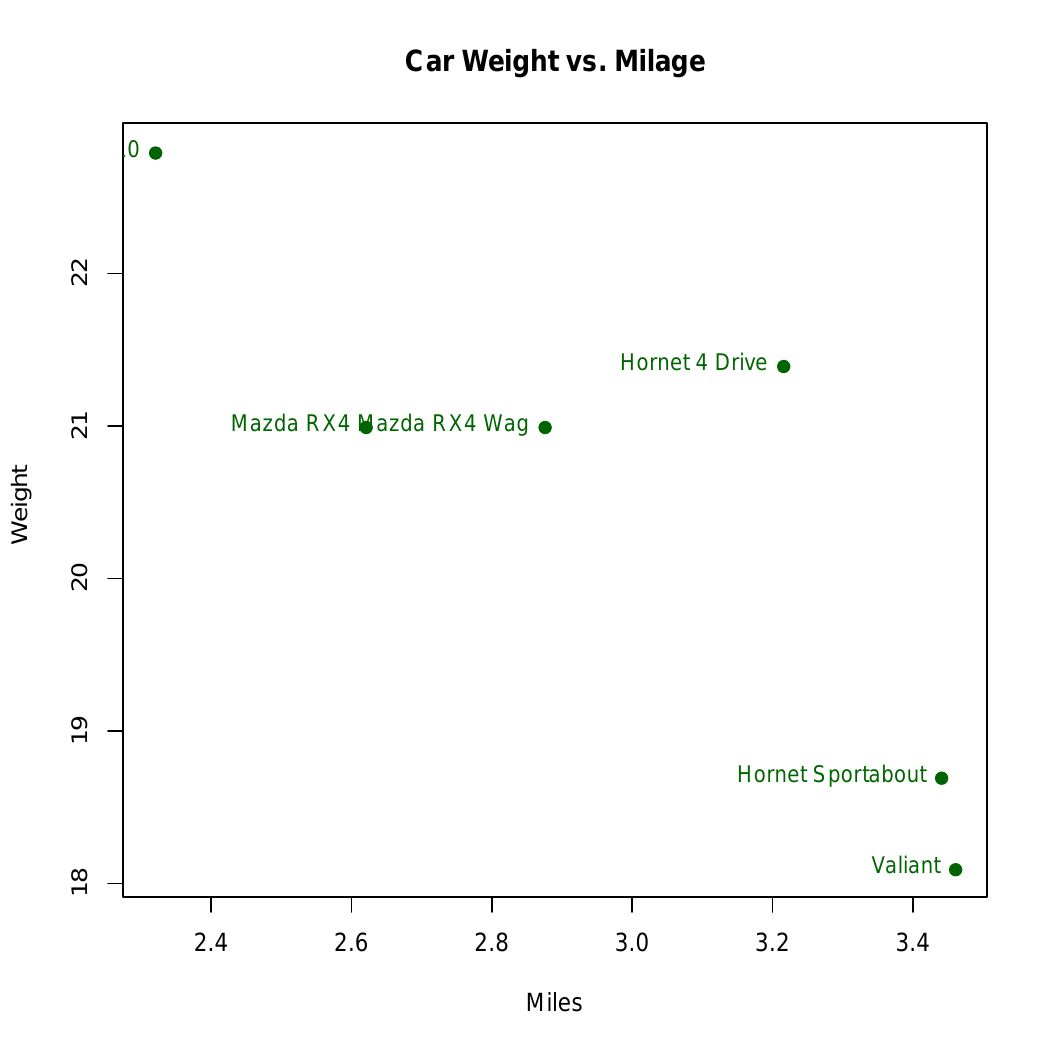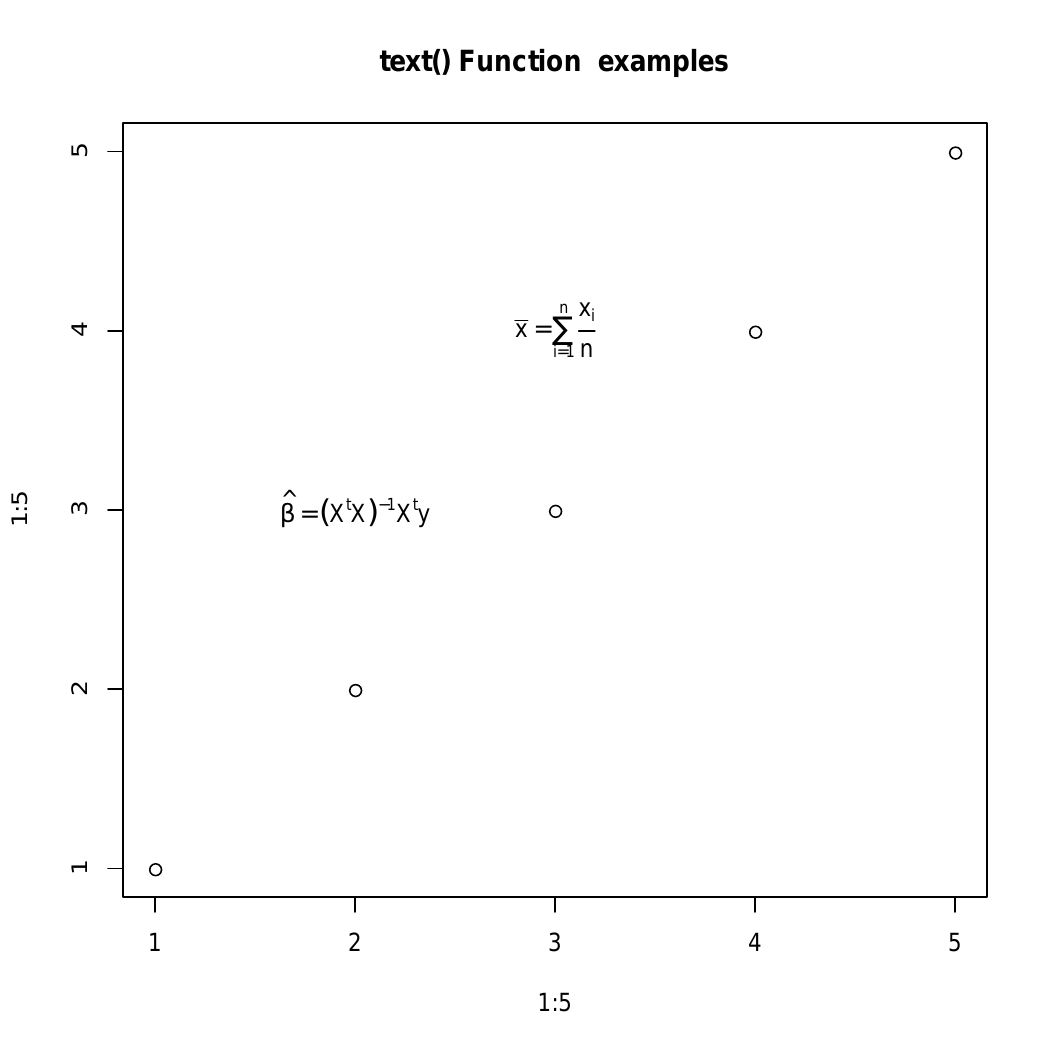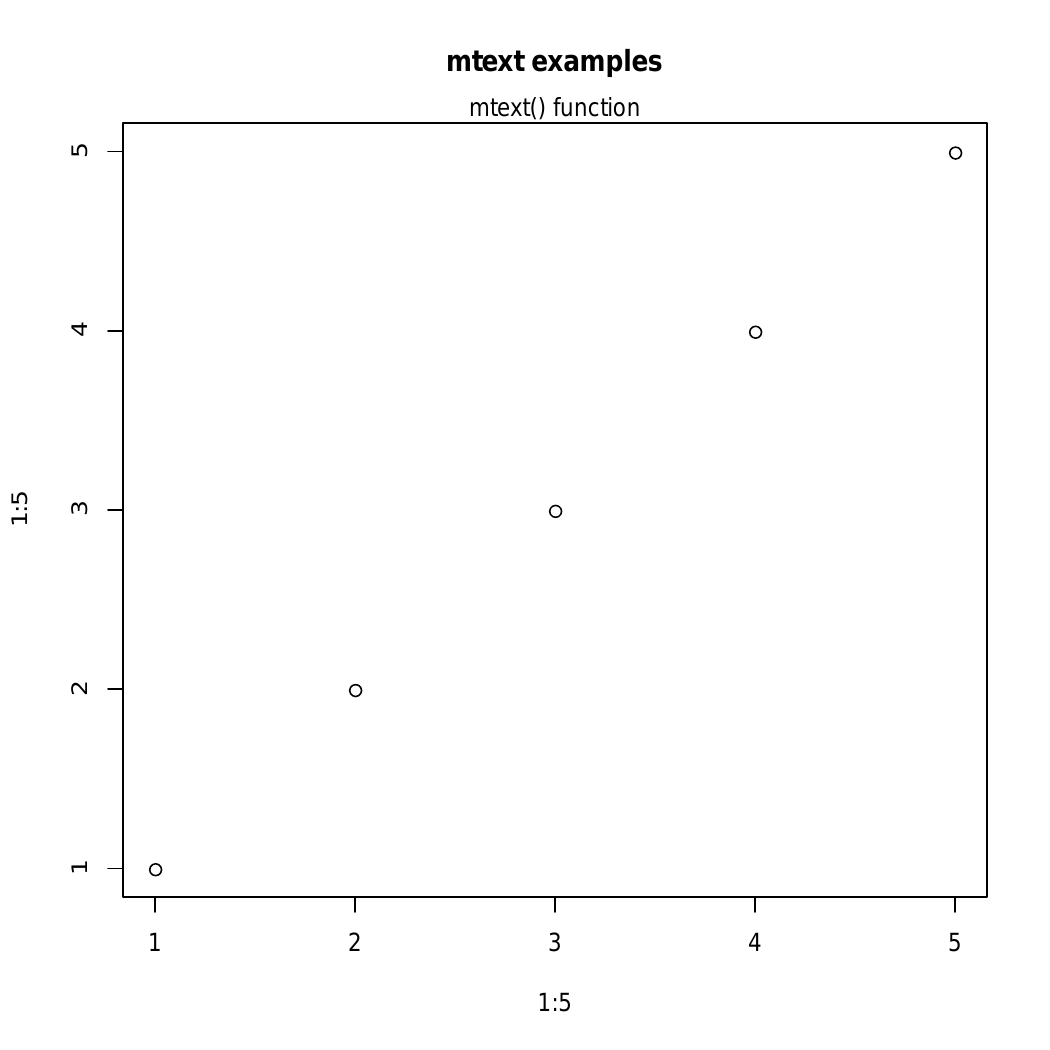Open in App
Not now

# Adding Text to Plots in R programming – text() and mtext () Function

• Last Updated : 07 Dec, 2021

Text is defined as a way to describe data related to graphical representation. They work as labels to any pictorial or graphical representation. In this article, we will learn to add a text to a plot in R Programming Language by using the text() and mtext() functions.

## R – text () Function

text () Function in R Programming Language is used to draw text elements to plots in Base R.

Syntax: text(x, y, labels)

Parameters:

• x and y: numeric values specifying the coordinates of the text to plot
• labels: the text to be written

Example 1:

## R

 `# R program to add text to plot` `# Calling data set``d<-``head``(mtcars)` `# Plotting the graph``plot``(d[, ``'wt'``], d[, ``'mpg'``],``     ``main = " Car Weight vs. Milage ",``     ``xlab = "Miles", ylab = " Weight",``     ``pch = 19, col = "darkgreen")` `# Calling text() function``text``(d[, ``'wt'``], d[, ``'mpg'``],  ``row.names``(d),``     ``cex = 0.88, pos = 2, col = "darkgreen")`

Output:In the above example, the text is added to the plot of ‘mtcar’ dataset.

Example 2: Implementation of text() to add a mathematical annotation to a plot

## R

 `# R program to add text to plot` `# Plotting the graph``plot``(1:5, 1:5,``     ``main = "``text``() Function  examples")` `# Calling text() function``text``(2, 3, ``expression``(``hat``(beta) == (X^t * X)^{-1} * X^t * y))``text``(3, 4, ``expression``(``bar``(x) == ``sum``(``frac``(x[i], n), i==1, n)))`

Output:In the above example, the text() function is used to add a mathematical annotation to a plot

## R – mtext () Function to Add text to the margins of the graph

mtext() function in R Programming Language is used to add text to the margins of the plot.

Syntax: mtext(text, side)

Parameters:

• text: text to be written
• side: An integer specifying the side of the plot, such as: bottom, left, top, and right.

Returns: Added text in the margins of the graph

Example:

## R

 `# R program to add text to a plot` `# Creating a plot``plot``(1:5, 1:5,``     ``main = "mtext examples")` `# Calling mtext() function``mtext``("``mtext``() ``function``", side = 3)`

Output:Here, in the above example, the side specifies the side of the plot such as the bottom, left, top, right. And in the given the side=3 i.e the top part of the plot.

My Personal Notes arrow_drop_up